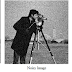## Analysis of denoised image using intensity profile

Image denoising is a process of recovering an original image from the noisy image using some denoising method/ filter. There are two ways of analyzing the denoised image i.e. quantitative analysis using metrics like PSNR, SSIM, UIQI, MSE etc. and qualitative analysis using visual assessment by naked eyes.
1. The above analysis methods are the traditional methods of image analysis which are highly effective.
2. There are some graph based assessment methods of denoised image that analyses either any particular line profile or the whole image.
3. There is one graph based assessment method i.e. intensity profile that compares the line profile of denoised image with the reference image.

### INTENSITY PROFILE (improfile)

1. Intensity profile is a line graph that plots the pixel values along the line segment of the image.
2. MATLAB provides an inbuilt function i.e. improfile that draws the intensity profile.
3. If the intensity profile of line segment of denoised image overlaps the intensity profile of line segment of reference image, then the denoising results are good.
4. And if the intensity profiles doesn't overlap, then the denoising results are not good.

### INTENSITY PROFILE (improfile) Implementation (MATLAB Code)

X = imread('cameraman.tif');     ---> X is the Reference Image
Y = imnoise(X,'gaussian',0,0.05);   ---> Y is the Noisy Image
Z = Denoising_Method(Y);   --->   Z is the Denoised Image
% Internsity Profile Overlapping
figure;
imshow(X);
improfile
hold on
figure;
imshow(Z);
improfile

Fig 1(a)                                                  Fig 1(b)                                       Fig 1(c)
Fig 2. Image with line profile
Fig 3. Overlapping of reference and denoised image
1. Fig 1(a) is reference image. Fig 1(b) is noisy image. Fig 1(c) is the denoised image.
2. Fig 2 is the image with line segment of which the intensity profile is calculated.
3. Fig 3. denotes the overlapping scenario of intensity profiles of reference and denoised images.
4. How to do Analysis: Since in the Fig 3. the intensity profiles of reference and denoised images are overlapping in a certain good way, that means the denoised image is almost similar to reference image i.e. denoising is satisfactory.
5. Note: This quality assessment technique can be used in any field of image restoration like digital image denoising, SAR image despeckling, medical image denoising etc.

For more image processing topics refer: https://www.gofastresearch.com/
Analysis of denoised image using intensity profileReviewed by IPR on April 19, 2020 Rating: 5